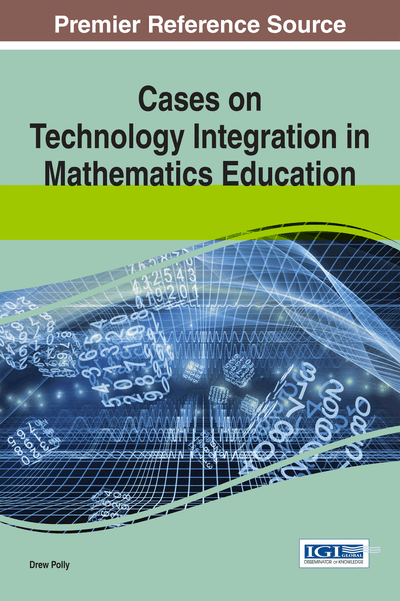# Utilizing Technology to Engage in Statistical Inquiry in Light of the Standards for Mathematical Practice

Christine Browning (Western Michigan University, USA) and Dustin Owen Smith (Western Michigan University, USA)
DOI: 10.4018/978-1-4666-6497-5.ch010

## Abstract

This chapter presents vignettes taken from a Probability and Statistics content course for elementary and middle school pre-service teachers. The vignettes are used to highlight issues when engaging students in statistical inquiry through technology use. Specifically, the authors address how particular Standards for Mathematical Practice should be understood differently from a perspective of statistical inquiry and how certain technological tools provide strong affordances for addressing these differences. The vignettes provide examples of what this could look like in a classroom by illustrating ways of attending to these practices in a statistical way through the use of technologies such as TinkerPlots®, Dropbox, Google Drive, and Interactive White Boards.
Chapter Preview
Top

## Introduction

Statistics is no more a branch of mathematics than is economics. . . . It is a separate discipline that makes heavy and essential use of mathematical tools, but has its origins, subject matter, foundational questions and standards that are distinct from those of mathematics. -David Moore, 1988

The distinction addressed by Moore (1988) between statistics and mathematics claims that the standards for these disciplines are (at least in some ways) distinct. How does this distinction impact how teachers of statistics should understand the Common Core State Standards for Mathematics? More specifically, to what extent are the Standards for Mathematical Practice also Standards for Statistical Practice? Thus, the goal of our chapter is to address these questions in a small way by providing vignettes that illustrate effective ways of using technology to teach statistics to middle school students to naturally allow for the development of particular Standards for Mathematical Practice (National Governors Association Center for Best Practices [NGACBP] & Council of Chief State School Officers [CCSSO]; 2010) that is compatible with the discipline of statistics. Additionally, these vignettes will serve as exemplars that illustrate how certain mathematical practices can be understood differently in light of the current research on the teaching and learning of statistics.

Top

## Technology And The Standards Of Mathematical Practice

As noted by the quote from Moore (1988), statistics is its own subject. And of all the courses mathematics teachers are typically asked to teach, statistics is the one in which their preparation is limited, with little emphasis on exploratory data analysis and the use of technology tools (Conference Board of the Mathematical Sciences, 2001). Thus we believe the teaching of statistics tends to take a more mathematical stance when taught from such a limited perspective. What we mean by that is that statistical problem solving in the classroom tends to take on an approach of “Given this data, find the mean, median, and mode” or “Make a box and whiskers plot of the given data and show the median and quartile values” or perhaps students may be challenged to find missing data values in a small set of data with given statistical constraints such as the values of the mean, median, and range. Such problem solving is not truly statistical in nature but more mathematical; statisticians generally don’t search for a missing set of data values to match given measures of center. “Statistics is the science of reasoning from data” (Rossman, Chance, & Lock, 2001, p 3) not computing with numbers in a way that is separated from any context. Data should be considered as numbers in a context and we should study these data with a purpose in mind (Rossman, Chance, & Lock, 2001). Students should engage in this purposeful inquiry with data while learning about statistics itself (Makar and Rubin, 2009) rather than in a sterile application of statistical measures.

Statistical visualization tools such as Fathom™ Dynamic Statistics (Key Curriculum Press, 2000) and TinkerPlots® (Konold & Miller, 2005), now exist that help students engage in the purposeful inquiry. These tools are not used for simply computing statistical measures but provide new ways for students to explore and analyze data, focusing on interpreting the data, perhaps even in ways that we as teachers have not even considered. The use of this type of technology then encourages a change in a teacher’s pedagogy, as the discourse around statistical concepts will change from a focus on computational mechanics to a focus on the legitimacy of student arguments regarding their final conclusions (Chance, Ben-Zvi, Garfield & Medina, 2007; Hammerman & Rubin, 2004).

## Complete Chapter List

Search this Book:
Reset